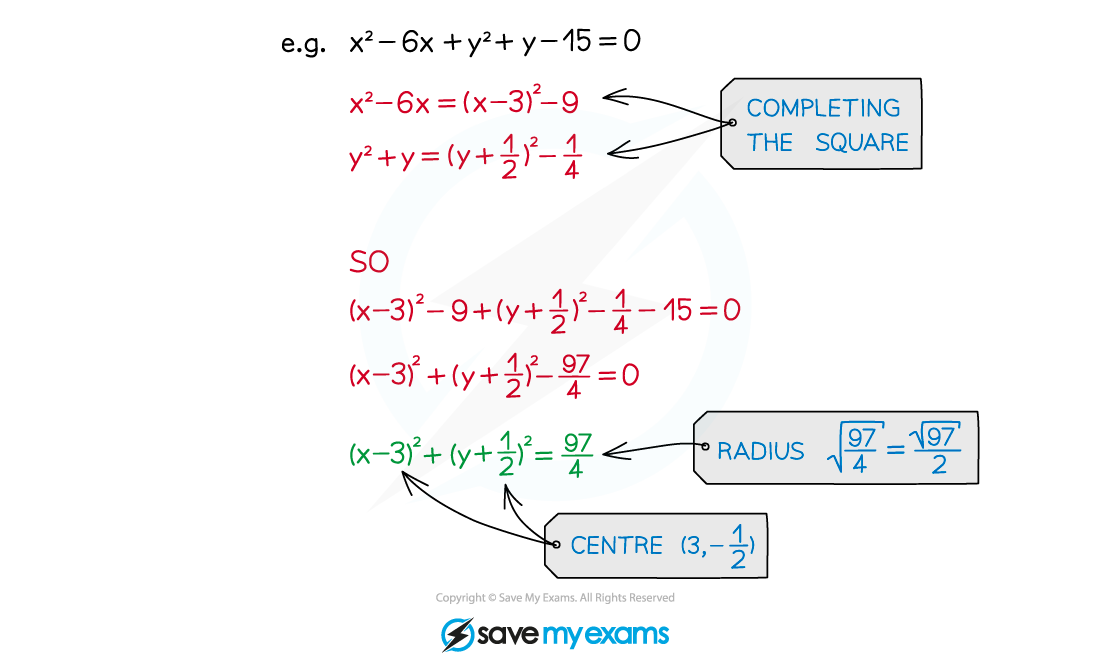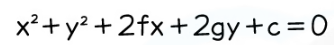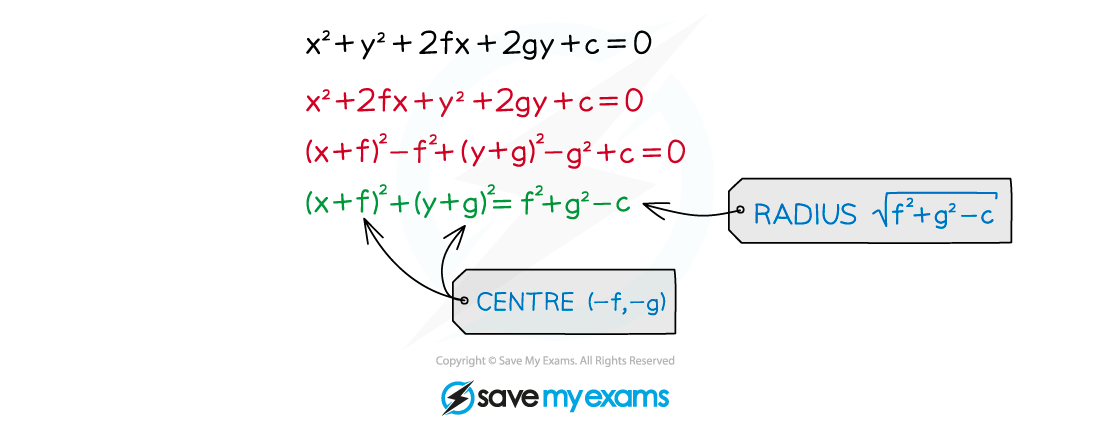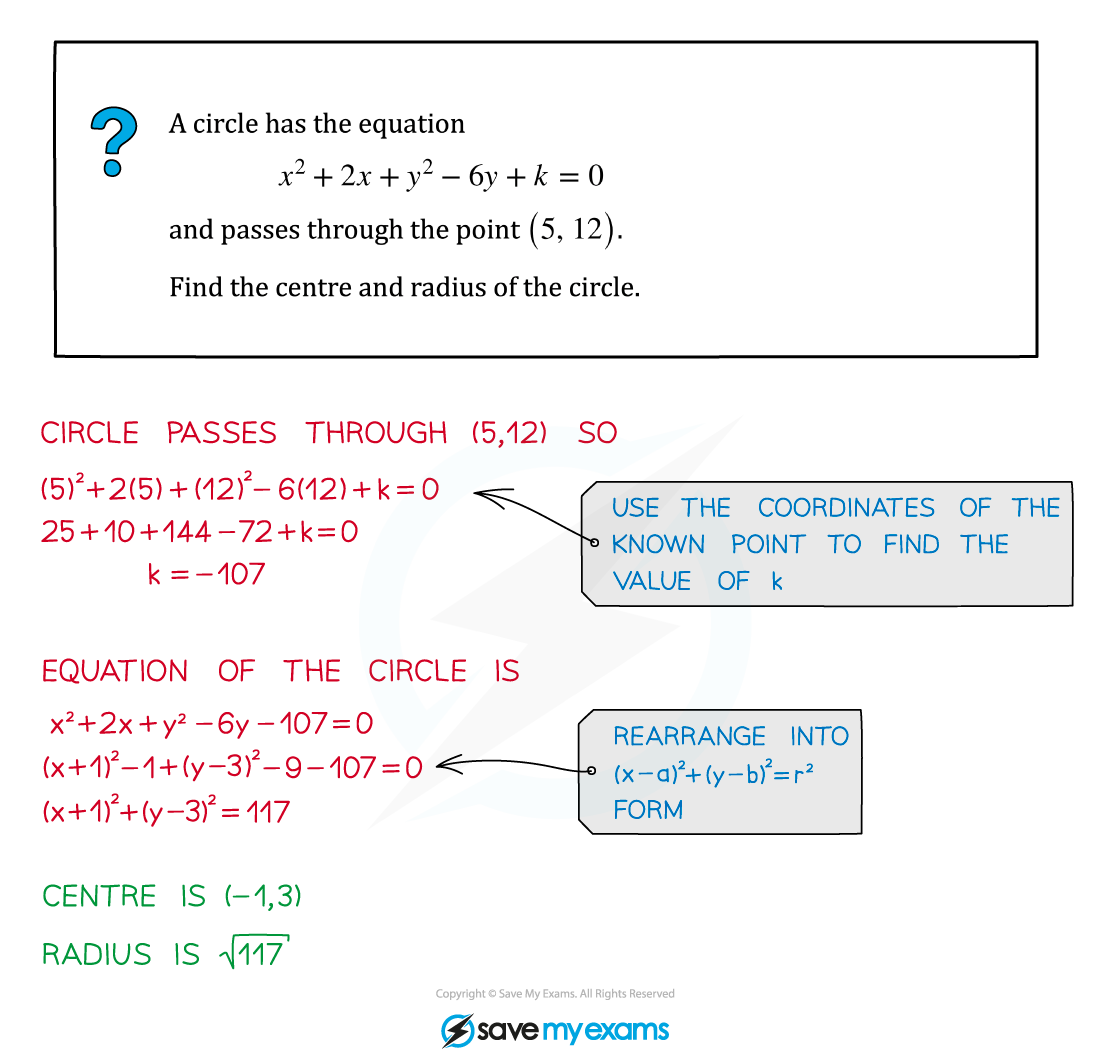# AQA A Level Maths: Pure复习笔记3.2.2 Finding the Centre & Radius

### Finding the Centre & Radius

#### How can I find the centre and radius from any form of the equation of a circle?

• A circle equation in a different form can always be rearranged into (x- a)2 + (y - b)2 = r2 form in order to find the centre and radius
• This will often involve completing the square#### Exam Tip

Make sure you are able to rearrange circle equations given in the general form#### Worked Example